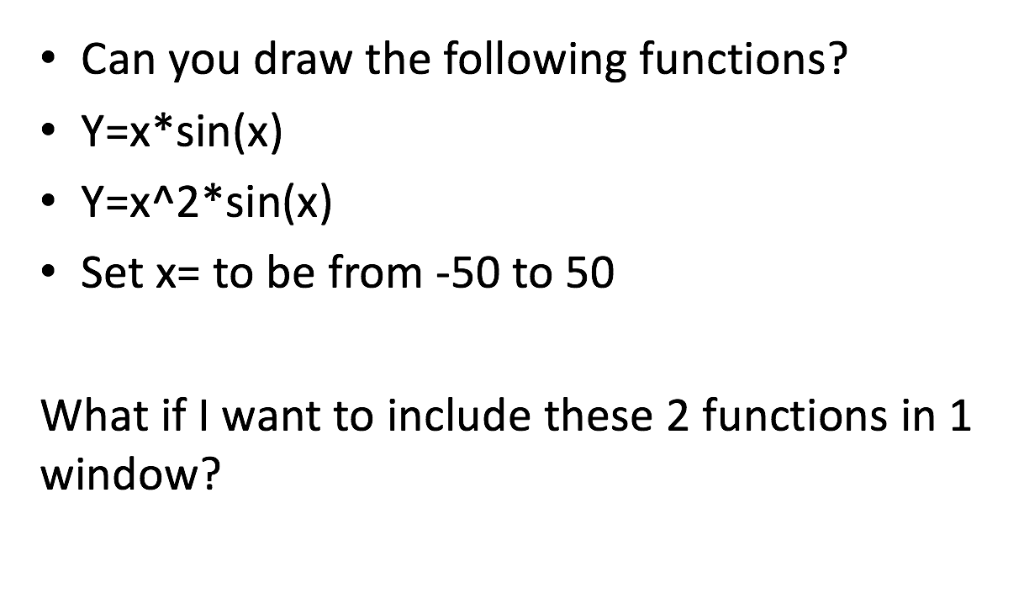Question

How can I do this on matlab? Steps would be greatly appreciated.Thanks. Can you draw the following functions? ·Y=x*sin(x) ·Y=x^2*sin(x) . Set x- to be from -50 to 50 What if I want to include these 2 functions in 1 window? Show transcribed image text . Can you draw the